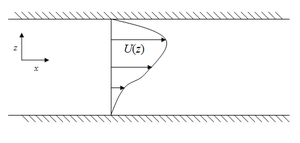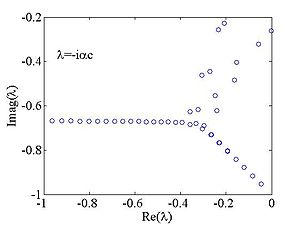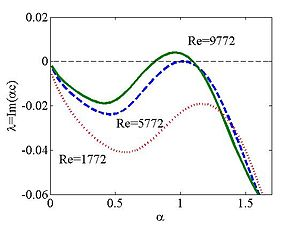# Orr–Sommerfeld equation

Orr–Sommerfeld equation

The Orr–Sommerfeld equation, in fluid dynamics, is an eigenvalue equation describing the linear two-dimensional modes of disturbance to a viscous parallel flow. The solution to the Navier–Stokes equations for a parallel, laminar flow can become unstable if certain conditions on the flow are satisfied, and the Orr–Sommerfeld equation determines precisely what the conditions for hydrodynamic stability are.

The equation is named after William McFadden Orr and Arnold Sommerfeld, who derived it at the beginning of the 20th century.

## FormulationA schematic diagram of the base state of the system. The flow under investigation represents a small perturbation away from this state. While the base state is parallel, the perturbation velocity has components in both directions.

The equation is derived by solving a linearized version of the Navier–Stokes equation for the perturbation velocity field$\mathbf{u} = \left(U(z)+u'(x,z,t), 0 ,w'(x,z,t)\right)$,

where (U(z),0,0) is the unperturbed or basic flow. The perturbation velocity has the wave-like solution$\mathbf{u}' \propto \exp(i \alpha (x - c t))$ (real part understood). Using this knowledge, and the streamfunction representation for the flow, the following dimensional form of the Orr–Sommerfeld equation is obtained:$\frac{\mu}{i\alpha\rho} \left({d^2 \over d z^2} - \alpha^2\right)^2 \varphi = (U - c)\left({d^2 \over d z^2} - \alpha^2\right) \varphi - U'' \varphi$,

where μ is the dynamic viscosity of the fluid, ρ is its density, and φ is the potential function. The equation can be written in non-dimensional form by measuring velocities according to a scale set by some characteristic velocity U0, and by measuring lengths according to channel depth h. Then the equation takes the form${1 \over i \alpha \, Re} \left({d^2 \over d z^2} - \alpha^2\right)^2 \varphi = (U - c)\left({d^2 \over d z^2} - \alpha^2\right) \varphi - U'' \varphi$,

where$Re=\frac{\rho U_0 h}{\mu}$

is the Reynolds number of the base flow. The relevant boundary conditions are the no-slip boundary conditions at the channel top and bottom z = z1 and z = z2,$\alpha \varphi = {d \varphi \over d z} = 0$ at z = z1 and z = z2.

The eigenvalue parameter of the problem is c and the eigenvector is φ. If the imaginary part of the wave speed c is positive, then the base flow is unstable, and the small perturbation introduced to the system is amplified in time.

## Solutions

For all but the simplest of velocity profiles U, numerical or asymptotic methods are required to calculate solutions. Some typical flow profiles are discussed below. In general, the spectrum of the equation is discrete and infinite for a bounded flow, while for unbounded flows (such as boundary-layer flow), the spectrum contains both continuous and discrete parts.The spectrum of the Orr–Sommerfeld for Poiseuille flow at criticality.Dispersion curves of the Poiseuille flow for various Reynolds numbers.

For plane Poiseuille flow, it has been shown that the flow is unstable (i.e. one or more eigenvalues c has a positive imaginary part) for some α when Re > Rec = 5772.22 and the neutrally stable mode at Re = Rec having αc = 1.02056, cr = 0.264002. To see the stability properties of the system, it is customary to plot a dispersion curve, that is, a plot of the growth rate Im(αc) as a function of the wavenumber α.

The first figure shows the spectrum of the Orr–Sommerfeld equation at the critical values listed above. This is a plot of the eigenvalues (in the form λ = − iαc) in the complex plane. The rightmost eigenvalue is the most unstable one. At the critical values of Reynolds number and wavenumber, the rightmost eigenvalue is exactly zero. For higher (lower) values of Reynolds number, the rightmost eigenvalue shifts into the positive (negative) half of the complex plane. Then, a fuller picture of the stability properties is given by a plot exhibiting the functional dependence of this eigenvalue; this is shown in the second figure.

On the other hand, the spectrum of eigenvalues for Couette flow indicates stability, at all Reynolds numbers. However, in experiments, Couette flow is found to be unstable to small, but finite, perturbations for which the linear theory, and the Orr-Sommerfeld equation do not apply. It has been argued that the non-normality of the eigenvalue problem associated with Couette (and indeed, Poiseuille) flow might explain that observed instability. That is, the eigenfunctions of the Orr–Sommerfeld operator are complete but non-orthogonal. Then, the energy of the disturbance contains contributions from all eigenfunctions of the Orr–Sommerfeld equation. Even if the energy associated with each eigenvalue considered separately is decaying exponentially in time (as predicted by the Orr–Sommerfeld analysis for the Couette flow), the cross terms arising from the non-orthogonality of the eigenvalues can increase transiently. Thus, the total energy increases transiently (before tending asymptotically to zero). The argument is that if the magnitude of this transient growth is sufficiently large, it destabilizes the laminar flow, however this argument has been criticized  since it invoques finite amplitudes in the context of a linear (that is zero amplitude) theory. A true explanation of instability in Couette and other shear flows such as Pipe and channel flows must include nonlinear effects.

A nonlinear theory ,  has been proposed instead. Although that theory does include linear transient growth, the focus is on elucidating the key 3D nonlinear process underlying transition and turbulence in shear flows. That nonlinear theory has led to the construction of complete 3D steady states, traveling waves and time-periodic solutions of the Navier-Stokes equations that capture many of the key features of transition and coherent structures observed in the near wall region of turbulent shear flows.      

For Couette flow, it is possible to make mathematical progress in the solution of the Orr–Sommerfeld equation. In this section, a demonstration of this method is given for the case of free-surface flow, that is, when the upper lid of the channel is replaced by a free surface. Note first of all that it is necessary to modify upper boundary conditions to take account of the free surface. In non-dimensional form, these conditions now read$\varphi={d \varphi \over d z}=0,$ at z = 0,$\frac{d^2\varphi}{dz^2}+\alpha^2\varphi=0$,$\Omega\equiv\frac{d^3\varphi}{dz^3}+i\alpha Re\left[\left(c-U\left(z_2=1\right)\right)\frac{d\varphi}{dz}+\varphi\right]-i\alpha Re\left(\frac{1}{Fr}+\frac{\alpha^2}{We}\right)\frac{\varphi}{c-U\left(z_2=1\right)}=0,$ at$\,z=1$.

The first free-surface condition is the statement of continuity of tangential stress, while the second condition relates the normal stress to the surface tension. Here$Fr=\frac{U_0^2}{gh},\,\,\ We=\frac{\rho u_0^2 h}{\sigma}$

are the Froude and Weber numbers respectively.

For Couette flow$U\left(z\right)=z$, the four linearly independent solutions to the non-dimensional Orr–Sommerfeld equation are,$\chi_1\left(z\right)=\sinh\left(\alpha z\right),\qquad \chi_2\left(z\right)=\cosh\left(\alpha z\right)$,$\chi_3\left(z\right)=\frac{1}{\alpha}\int_\infty^z\sinh\left[\alpha\left(z-\xi\right)\right]Ai\left[e^{i\pi/6}\left(\alpha Re\right)^{1/3}\left(\xi-c-\frac{i\alpha}{Re}\right)\right]d\xi,$$\chi_4\left(z\right)=\frac{1}{\alpha}\int_\infty^z\sinh\left[\alpha\left(z-\xi\right)\right]Ai\left[e^{5i\pi/6}\left(\alpha Re\right)^{1/3}\left(\xi-c-\frac{i\alpha}{Re}\right)\right]d\xi,$

where$Ai\left(\cdot\right)$ is the Airy function of the first kind. Substitution of the superposition solution$\varphi=\sum_{i=1}^4 c_i\chi_i\left(z\right)$ into the four boundary conditions gives four equations in the four unknown constants ci. For the equations to have a non-trivial solution, the determinant condition$\left|\begin{array}{cccc}\chi_1\left(0\right)&\chi_2\left(0\right)&\chi_3\left(0\right)&\chi_4\left(0\right)\\ \chi_1'\left(0\right)&\chi_2'\left(0\right)&\chi_3'\left(0\right)&\chi_4'\left(0\right)\\ \Omega_1\left(1\right)&\Omega_2\left(1\right)&\Omega_3\left(1\right)&\Omega_4\left(1\right)\\ \chi_1''\left(1\right)+\alpha^2\chi_1\left(1\right)&\chi_2''\left(1\right)+\alpha^2\chi_2\left(1\right)&\chi_3''\left(1\right)+\alpha^2\chi_3\left(1\right)&\chi_4''\left(1\right)+\alpha^2\chi_4\left(1\right)\end{array}\right|=0$

must be satisfied. This is a single equation in the unknown c, which can be solved numerically or by asymptotic methods. It can be shown that for a range of wavenumbers α and for sufficiently large Reynolds numbers, the growth rate αci is positive. Edit: the notation αc for the growth rate is not clear.

Wikimedia Foundation. 2010.

### Look at other dictionaries:

• SOMMERFELD — GERMANY (see also List of Individuals) 5.12.1868 Königsberg/RU 26.4.1951 Munich/D Arnold Sommerfeld read mathematics and physics at Königsberg University, formerly in Prussia and today s Kaliningrad in Russia. After two years as an assistant at… …   Hydraulicians in Europe 1800-2000

• Arnold Sommerfeld — Infobox Scientist box width = 300px name = Arnold Sommerfeld |250px image width = 250px caption = Arnold Johannes Wilhelm Sommerfeld (1868 1951) birth date = birth date|df=yes|1868|12|5 birth place = Königsberg, Province of Prussia death date =… …   Wikipedia

• William McFadden Orr — (2 May 1866 ndash; 14 August 1934) was an Irish mathematician.He was born in Comber, County Down and educated at Methodist College Belfast and Queen s College, Belfast before entering St John s College, Cambridge and graduating as Senior Wrangler …   Wikipedia

• Зоммерфельд, Арнольд — Арнольд Зоммерфельд Arnold Sommerfeld Зоммерфельд в …   Википедия

• Werner Heisenberg — Infobox Scientist box width = 300px name = Werner Heisenberg image size = 250px caption = Werner Karl Heisenberg (1901 1976). Photo taken circa 1926. birth date = birth date|1901|12|5|df=y birth place = Würzburg, Germany death date = death date… …   Wikipedia

• Gravity wave — In fluid dynamics, gravity waves are waves generated in a fluid medium or at the interface between two media (e.g. the atmosphere or ocean) which has the restoring force of gravity or buoyancy.When a fluid parcel is displaced on an interface or… …   Wikipedia

• Доказательные вычисления — Доказательные вычисления  целенаправленные вычисления на ЭВМ, комбинируемые с аналитическими исследованиями, которые приводят к строгому установлению новых фактов и доказательству теорем. Содержание 1 Достоверные вычисления 2 …   Википедия

• Гидродинамическая устойчивость — Развитие неустойчивости Кельвина Гельмгольца в атмосфере Сатурна Теория гидродинамической устойчивости  раздел гидродинамики и теории устойчивости, изучающий услов …   Википедия

• AÉRODYNAMIQUE — L’aérodynamique, dont l’étymologie évoque immédiatement l’action de l’air en mouvement, est la science qui étudie les différents aspects de cette action, notamment les forces, pressions et moments qui résultent du déplacement des corps dans… …   Encyclopédie Universelle

• Список научных публикаций Альберта Эйнштейна — Альберт Эйнштейн (1879 1955) был известным специалистом по теоретической физике, который наиболее известен как разработчик общей и специальной теорий относительности. Он также внёс большой вклад в развитие статистической механики, особенно… …   Википедия